# Poisson Distribution in Trefík

Predicting results using a statistical method

Software Trefik allows to use a lot of ways for matches analysis. One of them is using the Poisson distribution.

There are a lot of information about this method at Internet: wiki or Google.

Trefik allows using the Poisson distribution at the filter for the Offer window at the section Columns. You can check it directly inside Trefik: Filter / Samples: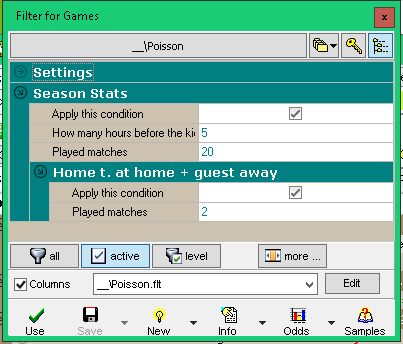.. the section Columns looks like this: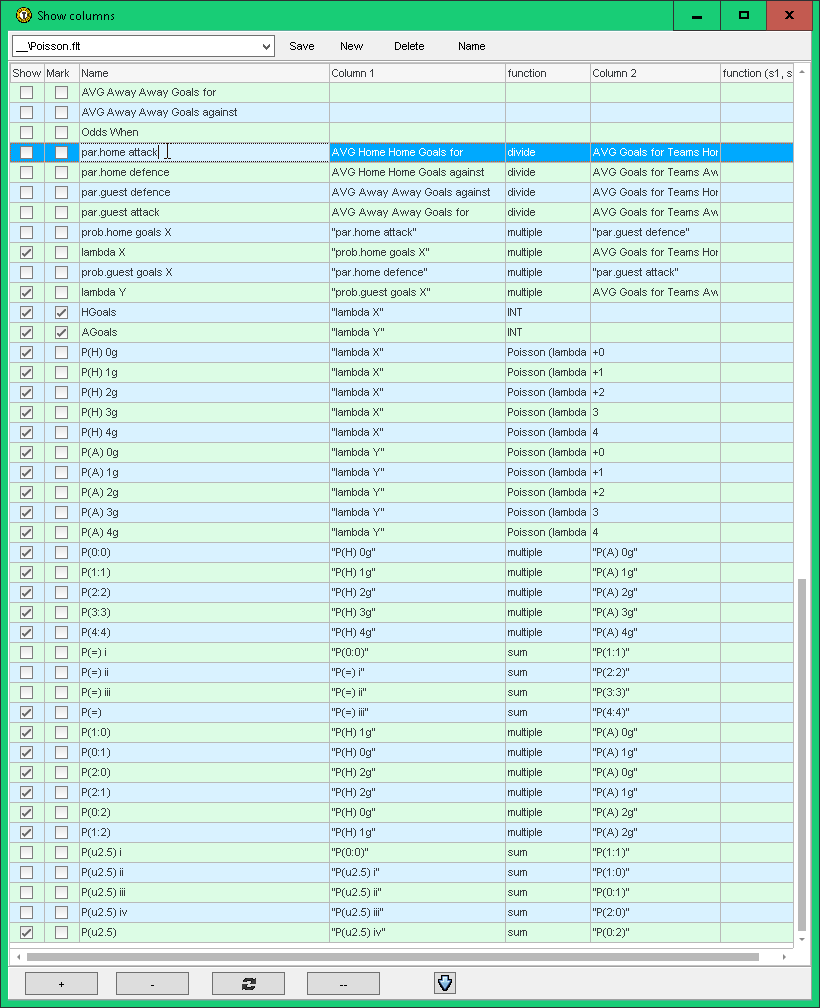.. the new columns are created for future counting and using the Poisson distribution.

First the parameters for attack and defence are counted for both teams. Then the probability for goals scored by both teams and also the lambda values for both teams (see the description links for the Poisson distribution).

We can use the counted values for creating new columns for analysing the matches:

• the estimated number of goals for both opponents,
• the probability of scoring the exact number of goals for both teams. For example "P(H) 0g" is the probability of the situation when the home team does not score any goal. "P(A) 3g" counts the probability of the situation when the guest team scores exactly 3 goals. The probabilities are counted using the internal function POISSON: its parameters are the previously counted value lambda and the set number of scored goals (the number of goals must be written with the + mark otherwise the columns would be exchanged for the odds for 1 or 2 bets),
• the probability of the exact result (it is equal to the sum of the probabilities of scoring the equivalent number of goals by both opponents),
• the probability of the result: the picture shows the calculation for the tie: as the sum of the probabilities of the exact results 0:0, 1:1, 2:2, 3:3 and 4:4,
• the probability of reaching the limit of scored goals (the picture partly shows the calculation for UNDER 2.5 goals).

When using that filter the Offer windows is like this: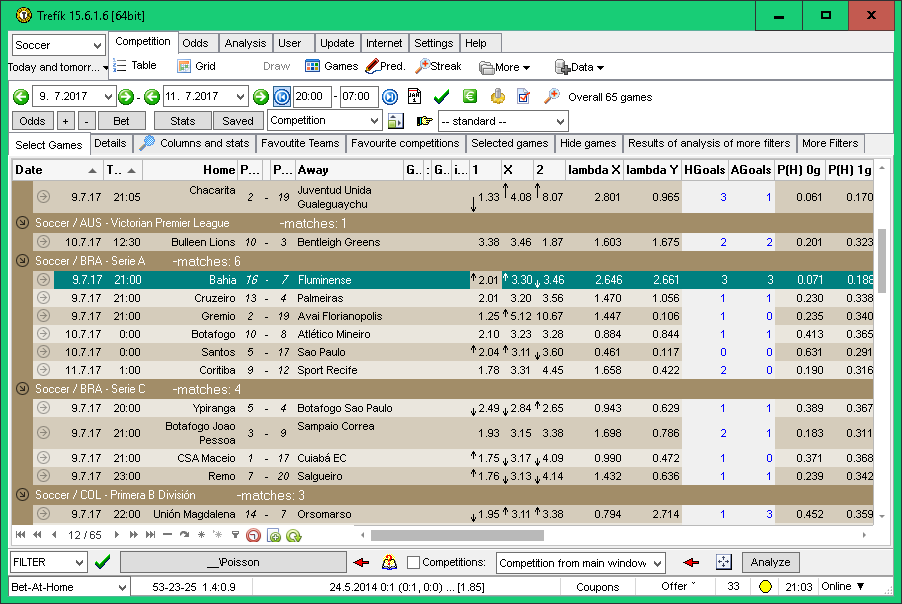.. and the next columns to the right: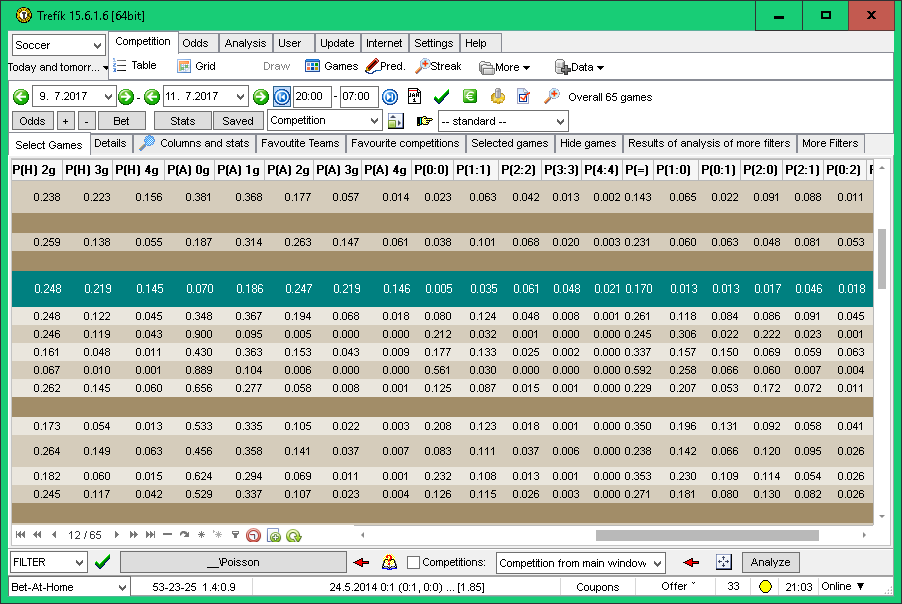.. and one more move to the right: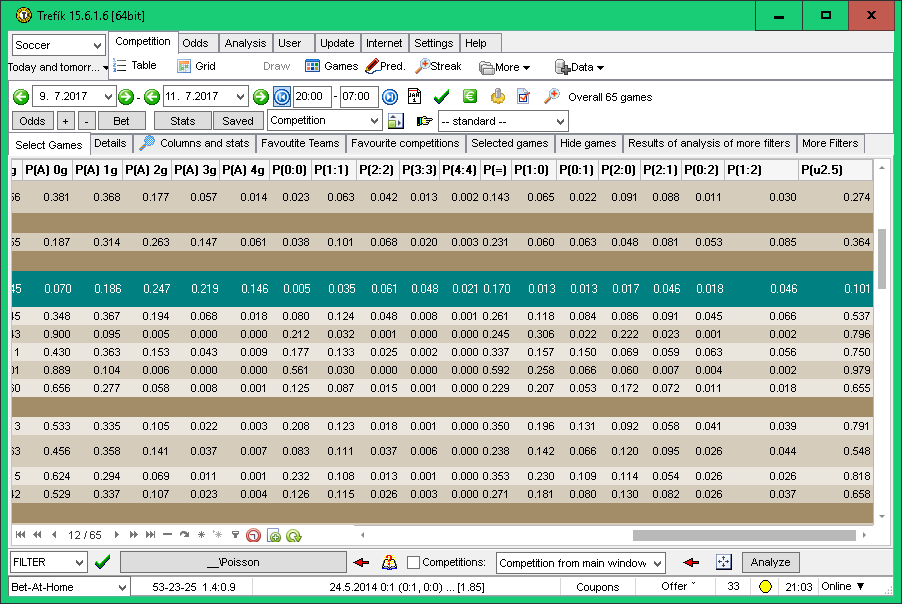.. the previous picture for example shows for the selected match the probability for scoring under 2.5 goals as only 10%.

The described filter can be used for already played matches and test the efficiency of the described method.